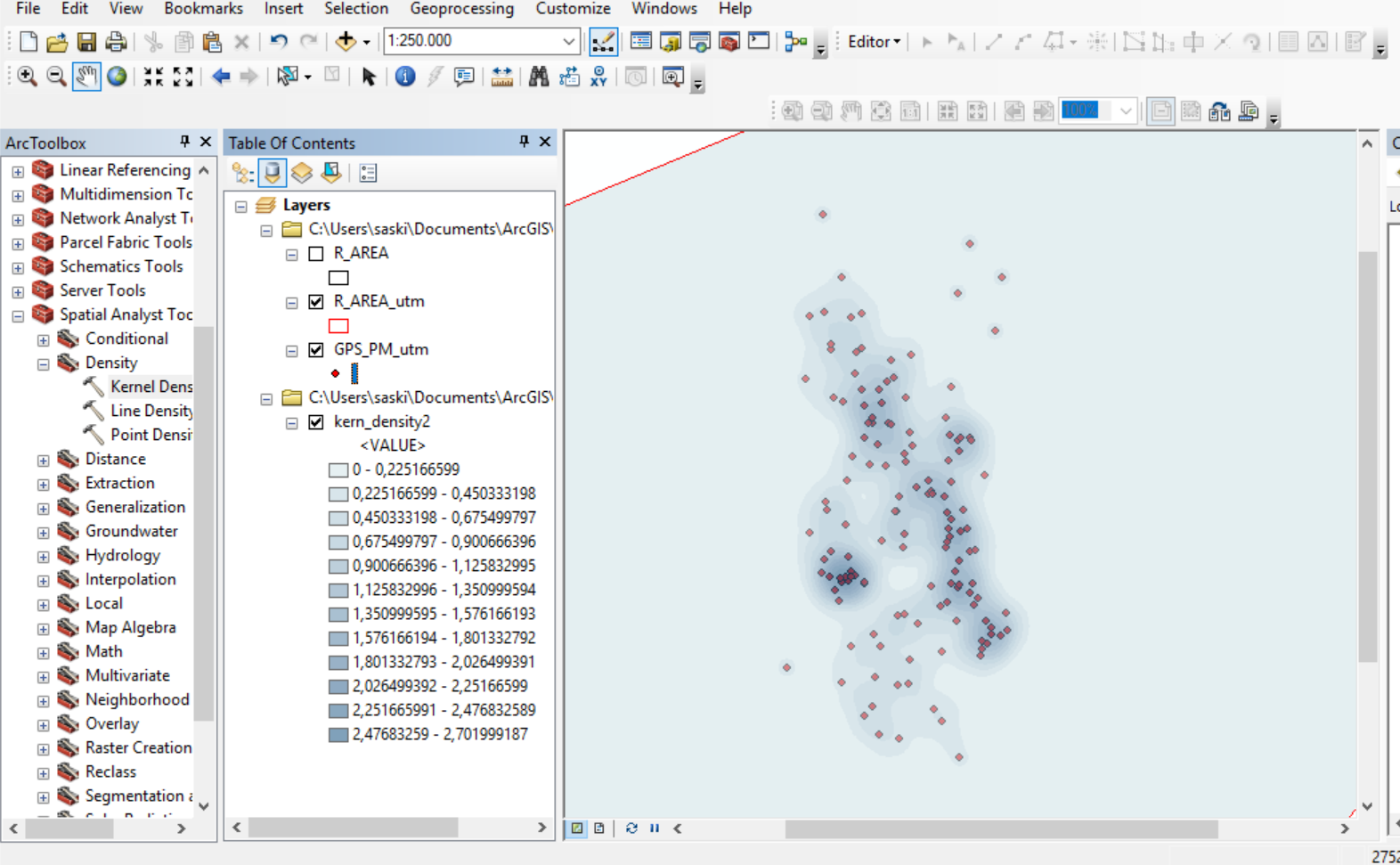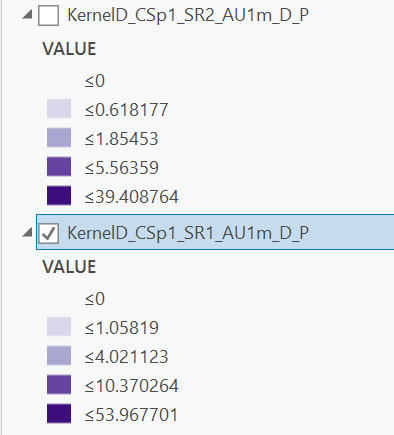# Value units kernel density?

1707
4
10-22-2018 05:55 AMNew Contributor II

I am trying to display the coordinates I collected of sperm whales I found during my research in Northern Norway, using the kernel tool to show the density within the area. General info: total amount of whales found 141, so 141 different coordinates, output cell size is 50 and area units default, so square kilometers.

The values I get from the kernel density are now between 0 - 2.7. Does that mean a maximum of 2.7 sperm whales per km² were found (so the highest aggregation on the map)? When I zoom in closer to the area where most whales were found, so between 2.4 - 2.7 according to the values, I count 5 individuals?

I read through earlier posts and other websites but couldn't find the answer I am looking for.

Thanks,Tags (6)
4 RepliesbyMVP Esteemed Contributor

I would confirm with the source

By default, a unit is selected based on the linear unit of the projection definition of the input polyline feature data or as otherwise specified in the output coordinate system environment setting.

When an output Area units factor is specified, it converts the units of both length and area. For example, if the linear unit is meters, the output area units will default to Square kilometers and the resulting line density units will convert to kilometers per square kilometer. The end result, comparing an area scale factor of meters to kilometers, will be the density values being different by a multiplier of 1,000.

You can control the density units by manually selecting the appropriate factor. To set the density to be in meters per square meter (instead of the default of kilometers per square kilometer), set the area units toSquare meters. Similarly, to have the density units of your output in miles per square mile, set the area units to Square miles.New Contributor II

Thanks for the quick reply. It still doesn't really answer my question though. I tried to set the area units to square meters but the only thing that happens is, that the numbers are now lower (eg 0.000027).byMVP Esteemed Contributor

ratios... if you found 1 object in 1 km^2 that is the same as having a density of 1/km^2 or 1/(1000*1000 m^2)… convert that ratio ...1/1e06m^2 = ?/1m^2Occasional Contributor

Hey Dan, I'm having the same trouble understanding the units of a kernel density.  The first output below is for 0.1 cell output size, 2 meter search radius, and area units square meters.  The second is the same except for using a 1 m search radius.  Symbology is quartiles for both.  I would expect the values to mean predicted points per unit of area, but then shouldn't I expect to see the values scale between the two outputs by the same value for each bin?